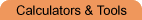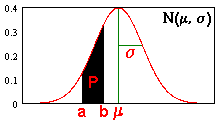Home    |    Teacher    |    Parents    |    Glossary    |    About UsEmail this page to a friendSelect Tool· Calculators · Converters · Equation Solvers · GraphersResources· Cool Tools · Formulas & Tables · References · Test Preparation · Study Tips · Wonders of MathSearchNormal Probability Calculator ( Math | Statistics | z-Distribution | Probability Calculator )

 Java Normal Probability Calculator (for Microsoft 2.0+/Netscape 2.0+/Java Script browsers only) To find the area P under the normal probability curve N(mean, standard_deviation) within the interval (left, right), type in the 4 parameters and press "Calculate". The standard normal curve N(0,1) has a mean=0 and s.d.=1. Use -inf and +inf for infinite limits.

 left bound right bound mean standard deviation